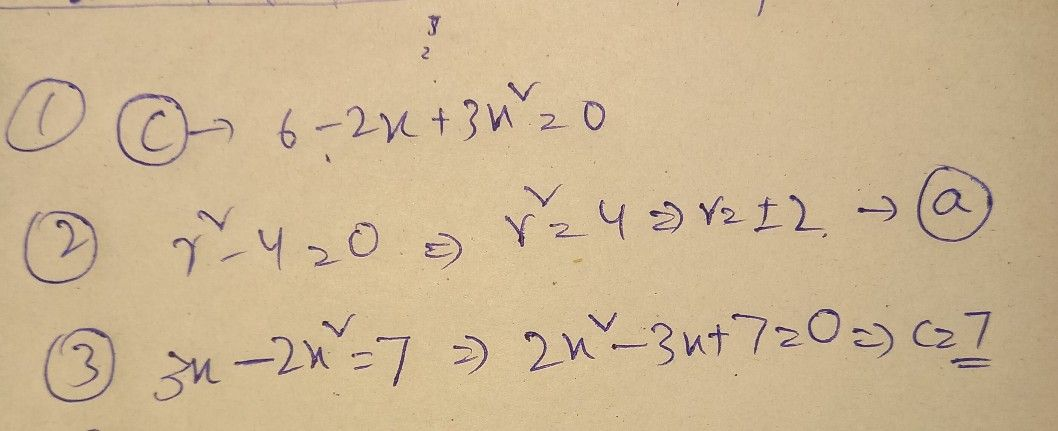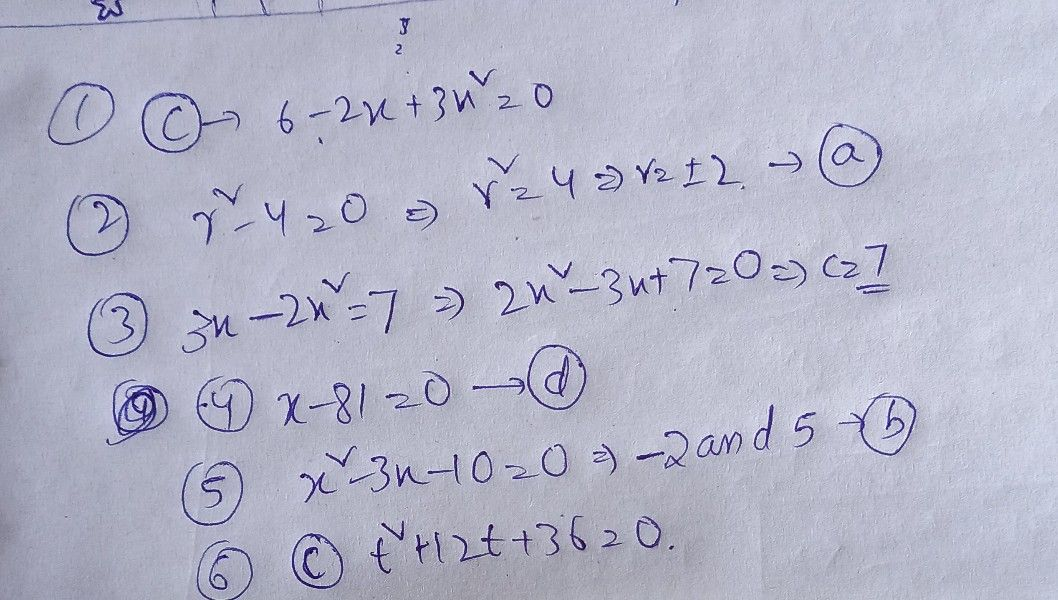Symbol
Problemthe correct answer on your answer sheet only the lem 1. Which of the following equations are quadratic? a. $3m+8=15$ $6-2x+3x^{2}$ =0 b. $25+3t=-7$ d. x+ y=C 2. Solve the quadratic equation by extracting square roots 7-40 a. 12 b. ±3 C14 d. 25 3. What is value of g in the equation of $3x-2x^{2}=n$ a. 3 b.7 C -7 d.2 4. Which of the following equations cannot bo solved by edracting squaro root? $x^{2}=9/16$ $0.$ $bx^{2}-7=0$ c.37 5. What are the roots of the $au0θ+$ equations x- $=18$ $d.x-81=0$ $-3x-10=0$ $a.10and1$ a. 2 and 5 $b-2and5$ $c-10and1$ 6. Which of the following quadratic equations may be solved more appropriately by $0eonn97$ $2x^{2}=7^{2}$ $b.w^{2}-64=0$ $cP+12+36=0$ $dx^{2}+2x=0$ $lcim0es9y$ a. 7. Find the solutions of the equation a. (3,6) $ionx^{2}+3x-18=0by$ $c\left(3,18\right)$ $d\left(0.3\right)$ completing square. 8. Find the solutions completing the square. $b.\left(1.3\right)$ $nsctn0$ $b.\left(1.3\right)$ equation x - $2x=3by$ $d.\left(0$ a. (3.-1) c. (-3, (0,3) 9. Find the solutions of the equation $2x^{2}+3x=27$ using the quadratic formula. a. 3 and - 9/2 b. 2 and 3 c 5 and 27 d. 2 and 9 10. What is the discriminant of the equation x+ 5x = 47 a. - 9/2 b3 c.5 d. 9 11. What is the nature of roots of the quadratic equation if the value of ts discriminant negative? roots are not real. c. tthhe e roots are roots irrational and not equal a. TThhe e are rational and not equal d. roots are rational and equal b. 12. Determine the nature of roots of the quadratic equation x + 6x +3 = 0. a. TThhe e roots are not arned al. not equal d. c tthhe e roots are roota irrational and nof aqual are rational roots are rational and equal
10th-13th grade
Algebra
Search count: 106
SolutionQanda teacher - Riya
any doubts feel free to ask dear